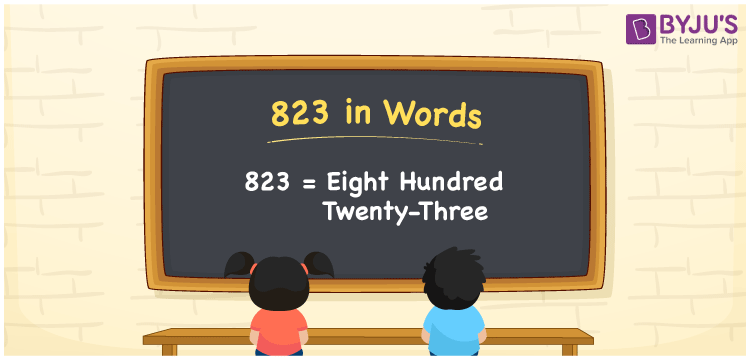# 823 in Words

We can write 823 in words as Eight hundred twenty-three. If you purchased a jokes book at Rs. 823, then you can say, “I purchased a jokes book for Eight hundred twenty-three rupees”. Here, Eight hundred twenty-three is the number name or word form for 823. In this article, you will understand how to write the cardinal number 823 in words using a place value chart and some facts about 823.

 823 in words Eight hundred twenty-three Eight hundred twenty-three in Numbers 823

## 823 in English Words

We generally write numbers in words using the English alphabet. Thus, we spell 823 in English words as “Eight hundred twenty-three”.## How to Write 823 in Words?

As we can see, 823 is a three-digit number so, let’s make a place value chart with three columns to convert 823 into words.

 Hundreds Tens Ones 8 2 3

Here, ones = 3, tens = 2, hundreds = 8

So, these numbers can be expanded as:

8 × Hundred + 2 × Ten + 3 × One

= 8 × 100 + 2 × 10 + 3 × 1

= 800 + 20 + 3

= Eight hundred + Twenty + Three

= Eight hundred twenty-three

Therefore, 823 in words is written as Eight hundred twenty-three.

823 is a natural number that precedes 824 and succeeds 822.

823 in words – Eight hundred twenty-three

Is 823 an even number? – No

Is 823 an odd number? – Yes

Is 823 a perfect square number? – No

Is 823 a perfect cube number? – No

Is 823 a prime number? – Yes

Is 823 a composite number? – No

## Frequently Asked Questions on 823 in Words

Q1

### How do you spell 823 in words?

We can spell the number 823 using English words as Eight hundred twenty-three.
Q2

### What is 0.823 in word form?

The word form of 0.823 is given as Eight hundred twenty-three thousandths (or) Zero point eight two three.
Q3

### Write an amount of Rs. 823 in words.

We can write an amount of Rs. 823 in words as “Eight hundred twenty-three in words”.